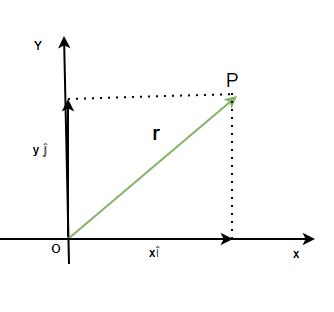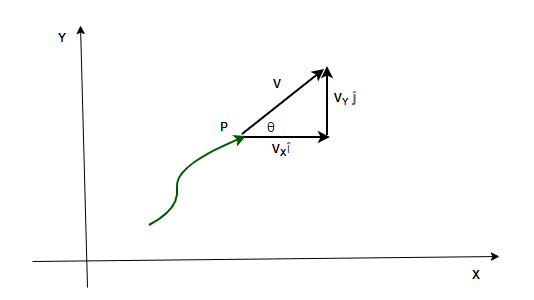Motion in Two Dimension

• Last Updated : 30 Jun, 2021

A particle moving in a plane can be described by using the coordinates. But often this information is not enough to fully describe the state and the behavior of the particle. There are still some questions left unanswered. For example, how fast is it moving? In which direction and what is the acceleration and direction of acceleration of the particle? These parameters are necessary to describe the motion of the particle in a 2D plane. Fortunately, all of this can be figured out using a bit of vector algebra and calculus. Let’s see how to do that in detail.

Motion in a Plane

Suppose a particle is moving from a point X on the Cartesian plane to a point Y. Position vectors are necessary to describe the current position of the particle. These vectors are always with respect to the reference frame at the origin. The following parameters are required to fully describe the behavior of a particle moving in a plane,

1. Position
2. Velocity
3. Acceleration

Position Vector

The vector which denotes the position and direction of the particle’s position with respect to the origin is called the position vector. The position vectorfor a particle is given by,Where x and y are their components along the x and y-axis.Velocity

The velocity of a particle can be described in two ways – average velocity and instantaneous velocity. When the particle is under acceleration, it changes its velocity every second. So, a single value cannot be assigned to a velocity. In such cases, the instantaneous velocity is preferred, which describes the velocity and its direction at a particular instant. It is given by,Velocity can also be expressed in the form of its components.The average velocity is the ratio of total displacement over total time. Suppose a particle goes fromtoin a total time ofThe velocity is given by,Acceleration

The acceleration of a body moving in a plane is given by the rate of change in its velocity. Similar to velocity, there can be two cases here too – average acceleration and instantaneous acceleration. Average acceleration is given by the ratio of the net change in the velocity of the object with the total time taken. Let initial and final velocities be denoted byandInstantaneous acceleration is used when the acceleration of the body is changing with time.This can also be decomposed into its components.The magnitude of acceleration can also be calculated using the components of velocity,And the direction is given by angle,Sample Problems

Question 1: Find the velocity at t = 2, for the particle which is moving in a plane and whose position is given below,

r = t2i + t2j

Given: the initial and final position vectors,

r = t2i + t2j

The position vector changes with time. The velocity in this case is given by the formula,Here x(t) = t2 and y(t) = t2

Plugging these values into the equation,At t = 2,Question 2: Find the velocity at t = 0, for the particle which is moving in a plane and whose position is given below,

r = (t+2)i + (4t2+2)j

Given: the initial and final position vectors,

r = (t+2)i + (4t2+2)j

The position vector changes with time. The velocity in this case is given by the formula,Here x(t) = t+2 and y(t) = 4t2+2

Plugging these values into the equation,at t = 0,Question 3: Find the average acceleration between t = 0 and t = 3, for the particle which is moving in a plane and whose position is given below,

v = 3ti + 3t3j

Given: velocity as a function of time.

v = 3ti + 3t3j

The velocity vector changes with time. The average acceleration is given by the formula,At t = 0

vi = 0i + 0j

At t = 3

vf = 9i + 81jPlugging the values into the above equation,Question 4: Find the average acceleration between t = 0 and t = 2, for the particle which is moving in a plane and whose position is given below,

v = ti + 3tj

Given: velocity as a function of time.

v = ti + 3tj

The velocity vector changes with time. The average acceleration is given by the formula,At t = 0

vi = 0i + 0j

At t = 2

vf = 2i + 6jPlugging the values into the above equation,Question 5: Find the instantaneous acceleration at t = 1, for the particle which is moving in a plane and whose position is given below,

r = ti + tj

Given: the initial and final position vectors,

r = ti + tj

The position vector changes with time. The acceleration in terms of position given by,x(t) = t and y(t) = t

Plugging the values into the equation,Question 6: Find the instantaneous acceleration at t = 1, for the particle which is moving in a plane and whose position is given below,

r = 3ti + t3j

Given: the initial and final position vectors,

r = 3ti + t3j

The position vector changes with time. The acceleration in terms of position given by,x(t) = 3t and y(t) = t3

Plugging the values into the equation,at t = 1

a = 6j

Question 7: A body is moving in a 2D plane, its acceleration along the x-axis is 4m/s2 and along the y-axis, it is 3m/s2. Find the magnitude and the angle of the acceleration on the x-axis.

The magnitude of acceleration can also be calculated using the components of velocity,ax = 4 m/s2 and ay = 3 m/s2

Plugging the values in the equation,The angle can be calculated using the equation,My Personal Notes arrow_drop_up